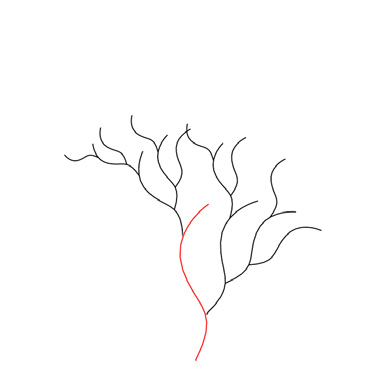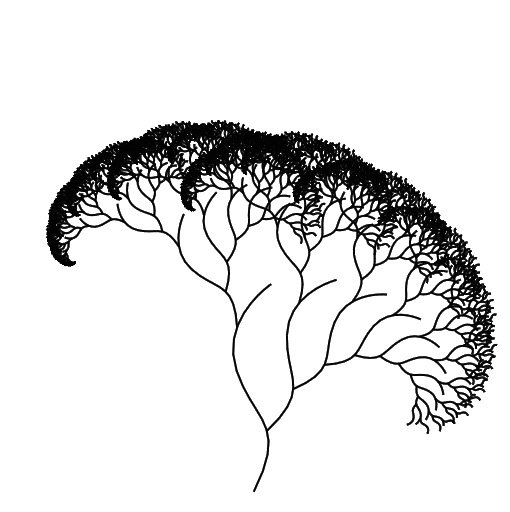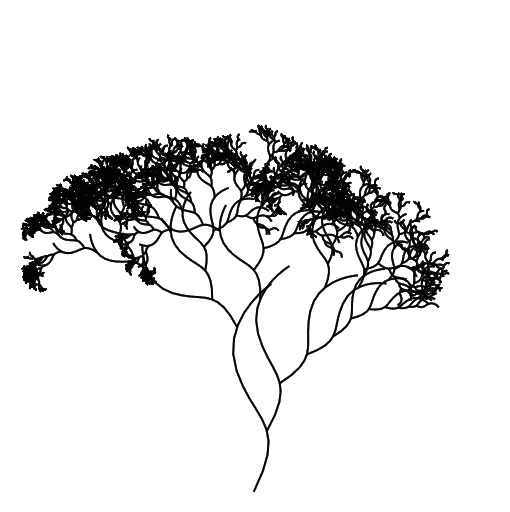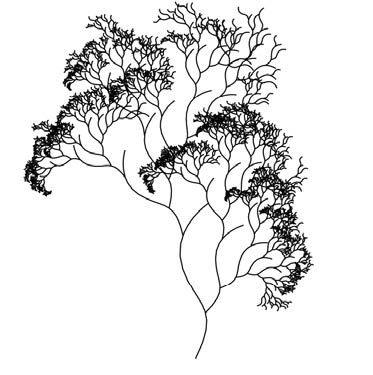Inverse Procedural Modeling by Automatic Generation of L-systems

Peter KryštofPurdue University

Goal

In this project we explored the possibilities of creating procedural rules from a given structure or pattern.

The key challenge of procedural techniques is the definition of the rules. This task is not intuitive, lacks controllability, requires in-depth knowledge of procedural modeling, and resembles programming in an advanced language. Automatic generation of procedural rules, or inverse procedural
modeling, has been an open problem for more than 20 years. Its goal is to find the rules of a procedural system that would generate a given model. Applications of such an automatic system would be immense. For example, changing the rules allows generation of classes of similar models, the internal structure of the model could be modified, the model could be represented by its generative rules (compressed), the syntactic analysis of the rules could be used for image analysis, and so forth.

Our approach

We made an important step towards the solution of the problem of inverse procedural modeling by generating parametric context-free L-systems that represent an input 2D model. The L-system rules efficiently code the regularstructures and the parameters represent the properties of the structure transformations.

The algorithm takes as input a 2D vector image that is composed of atomic elements, such as curves and poly-lines. Similar elements are recognized and assigned terminal symbols of an L-system alphabet. The terminal symbols’ position and orientation are pair-wise compared and the transformations are stored as points in multiple 4D transformation spaces. By careful analysis of the clusters in the transformation spaces, we detect sequences of elements and code them as L-system rules. The coded elements are then removed from the clusters, the clusters are updated, and then the analysis attempts to code groups of elements in (hierarchies) the same way. The analysis ends with a single group of elements that is coded as an L-system axiom. We recognize and code branching sequences of linearly translated, scaled, and rotated elements and their hierarchies.

The L-system not only represents the input image, but it can also be used for various editing operations. By changing the L-system parameters, the image can be randomized, symmetrized, and groups of elements and regular structures can be edited. By changing the terminal and non-terminal symbols, elements or groups of elements can be replaced.

System pipeline1. Similar elements of the input image are detected and terminal symbols are generated for each group of similar elements.
2. Pairwise transformations are calculated and transformation spaces are filled with points corresponding to each transformation. Mean shift clustering determines clusters of similarly oriented elements.
3. Cluster significance is calculated based on user-defined criteria.
4. Groups of elements are represented as L-system rules. Each group is represented as a new non-terminal and the clusters in the transformations space that contain references to them are updated.

The process ends with a single element that represents the axiom of the L-systema)b)c)d)

Figure 1: a) A branching structure with varying scaling is automatically coded as an L-system. The basic element is a Bézier curve (in red), the generated rule has form R(m)→A[−(17) f (64)∗(0.8)−(3)R(m−1)] [+(7) f (181)∗(0.6)+(23)R(m−1)], and the axiom is R(3). b) A structure generated using the detected rule from axiom R(10) and c) a branching structure generated from R(10) with randomized angles and d) randomized angles and scaling.

Project Publications

Šťava, O., Beneš, B., Měch, R., Aliaga, D., Krištof, P. (May. 3, 2010)
Computer Graphics Forum (Proc. Eurographics), 29(2), 2010